# php tutorial - PHP For Loop - php programming - learn php - php code - php script

• For loop statement is typically used to execute a block of code for certain number of times.
• for loop allows the user to put all the loop-related statements (which is INITIALIZER; CONDITION; INCREMENTOR or DECREMENTOR) in one place, which is similar to C language.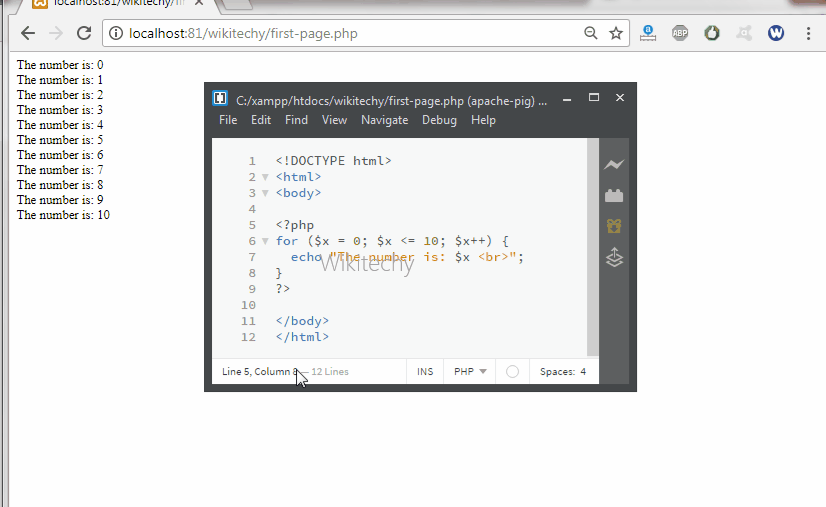Learn PHP - PHP tutorial - For loop - PHP examples - PHP programs

## Syntax Explanation :

• initialization: It is used to initialize the counter variables.
• test counter: Evaluate for each loop iteration. If it evaluates to be TRUE, the loop continues. If it evaluates to be FALSE, the loop ends.
• increment : It increase the loop counter with a new value. It is evaluating at the end of each iteration.
• execute the statement: It executes the php statements.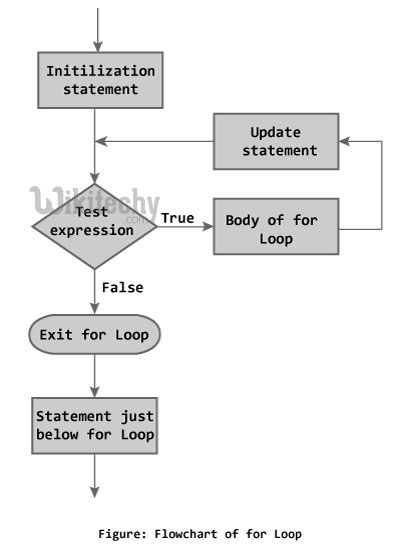## learn php Sample Code :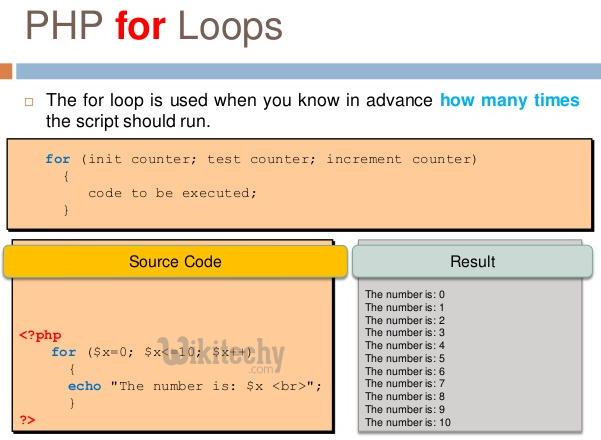## php for beginners Code Explanation :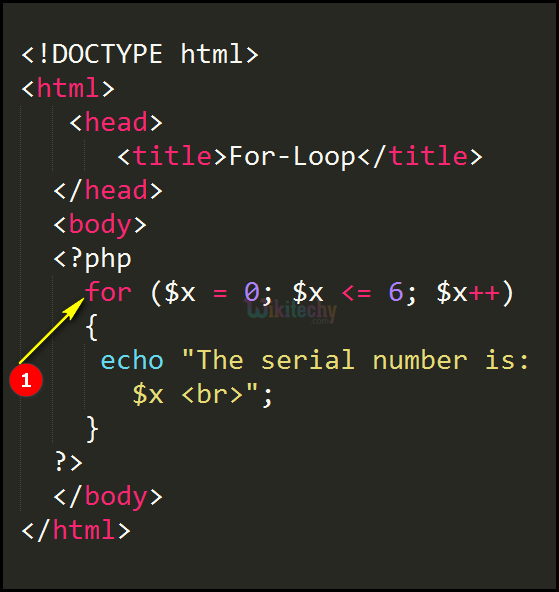1. Here for (\$x = 0; \$x <= 20; \$x++) specifies \$x = 1, which means the expression, (\$x<= 20), is true. Therefore, the echo statement is executed, and \$x gets incremented by 1 and becomes 2.\$x = 2, which means the expression, (\$x <= 20), is true. Therefore, the print statement is executed till the value hits 20, and \$x gets incremented by 2 and becomes 3.

## php coding Sample Output :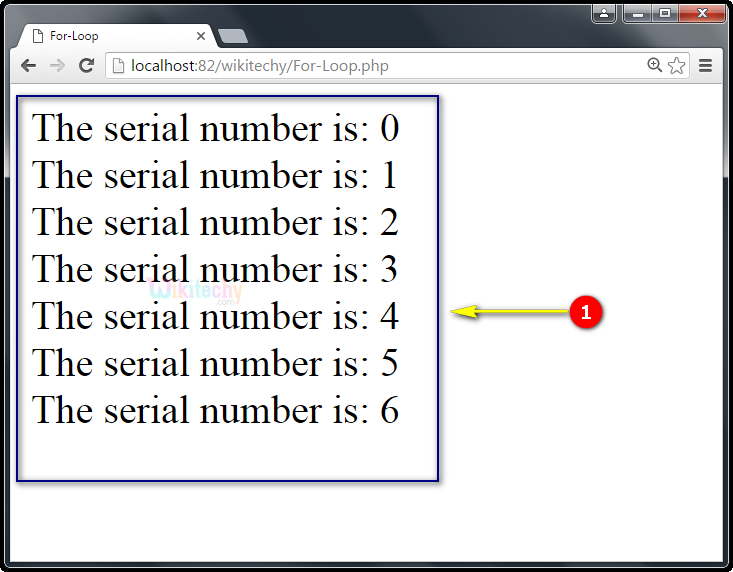1. Here in this output the serial numbers : 0,1,2,3,4,5,6 will be printed until it reaches the last element “6”.